Rese rch 1 . Solutions & Ideas .
Share this Site ' D N A ' if you like it .

' Assuming one Infinity, one Time is just a little grain of Sugar . '

Everything can be slighter by production without poisoning and new planetary exploitation
with a strong recommendation to recycle and to clean from industrial toxics ,
and to take care for each other Living ! Stop ( y ) our war and helping on the way ?

4. The Big Bang Theory ,
Gravitational Waves ,
Time Travelling of Antimatter and
its Smallest Circular Flow through space ( Magnetism ) ,
the Natural Curvature of Space and Time ,
a Hubble Parameter &
The Expansion Speed of Space within our Universe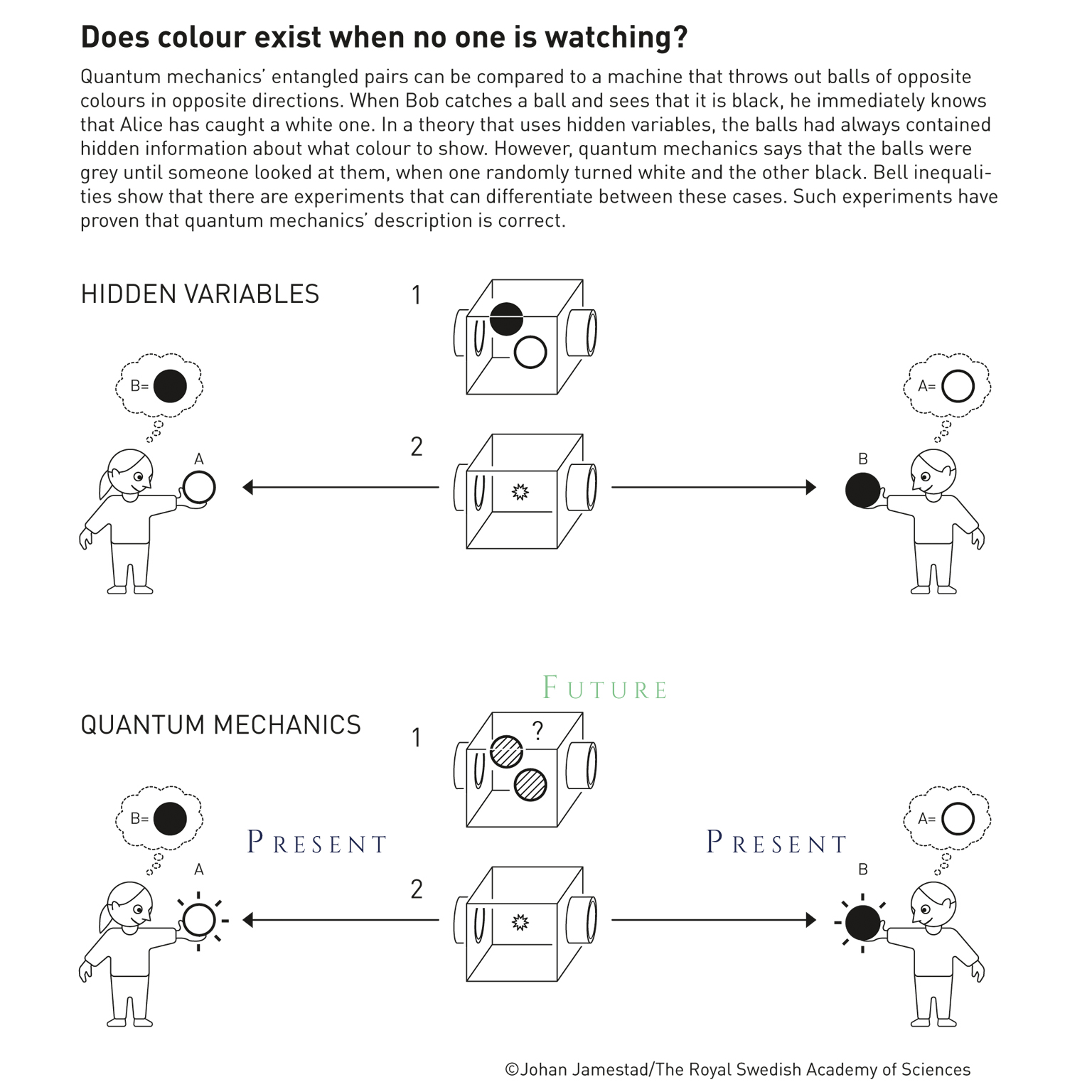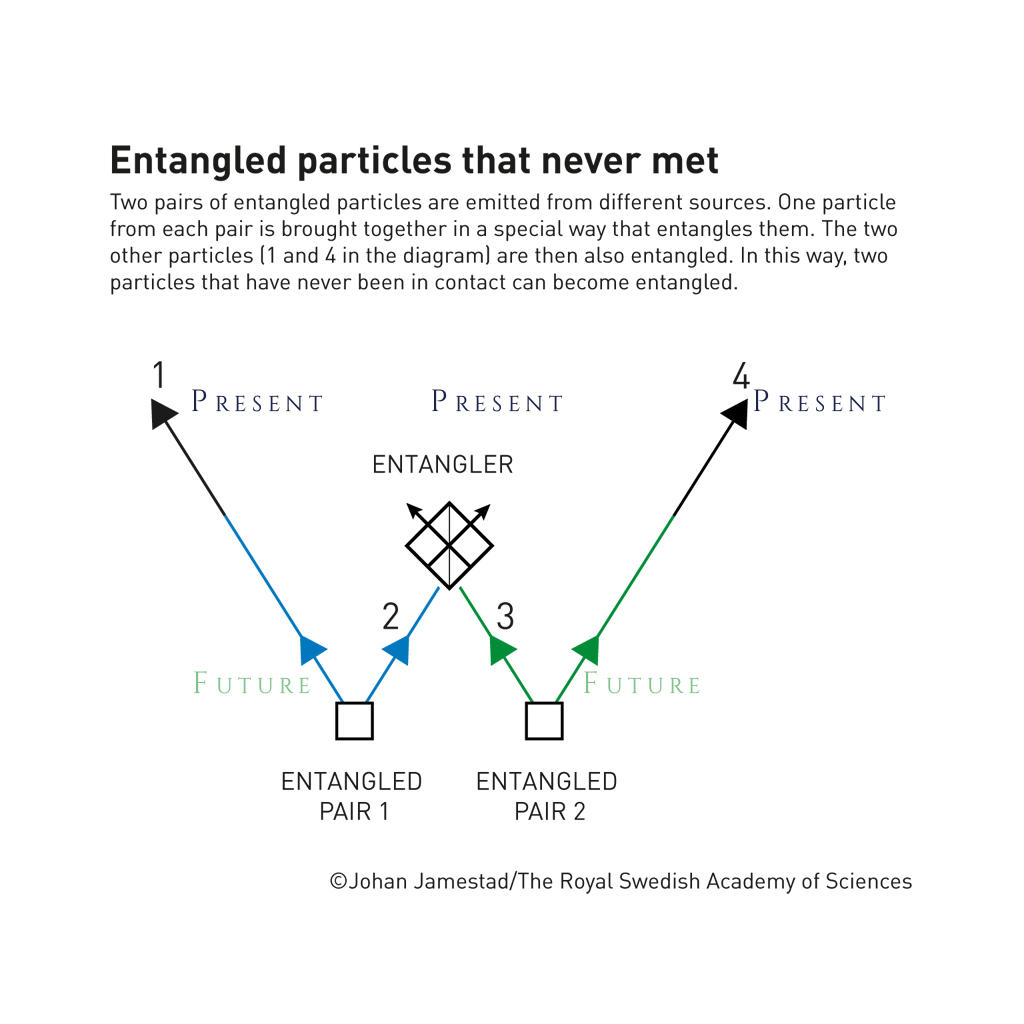Moving backward through time is kind of a ' spooky action ' , like
Albert Einstein has referred to as when having watched the phenomenon
of Quantum Teleportation by the behaviour of entangled photons ...
We are able to reach each point within time by speeds !
Moving through the three dimensions of space by speed is possible ,
whereas speed is measured in metres per time units , and hence
resulting metres per metres exponent 4 .
So we might move throughout time by metres squared per metres exponent 5 ,
what results metres per metres exponent 4 , equal to usual speeds .
' By Chance ' should not exist in classic physics nor in Quantum Mechanics ...
And present is , when Future meets Past !

We might have found to a logical proof for
Antimatter moving from future to past and
we start right ahead with these few lines :

( 1 ) = possible
( - 1 ) = impossible = entangled photons which never met
while this is only possible ;
( - 1 ) = impossible = moving from future to past ( our clocks move ' forward ' )

We are going to see that
( - 1 ) times ( - 1 ) = ( 1 ) = possible
and that
( 1 ) times ( 1 ) = ( 1 )
while
( 1 ) can be the logical inversion of ( - 1 ) also ...

Now let us see why we multiply both the factors :

An apple and a cake is an apple and a cake .
An apple times a cake is an ' applecake ' .
While an apple describes the entanglement
of photons which never met and the cake is
our clock moving backward ...

We might explain the multiplication by this way too :
( 1 / a ) plus ( 1 / c ) cannot be simplified more than
( 1 c / ac ) plus ( 1 a / ac ) =
( 1 c plus 1 a ) / ac
but what we can do is
( 1 / a ) times ( 1 / c ) = 1 / ac

1 / 4 of 1 is 1 / 4
' of ' can be described as ' times '
1 / 4 times 1 is 1 / 4
' impossible ' of ' impossible ' is ' possible '
so
' impossible ' times ' impossible ' is ' possible '

We would like to strengthen our proof for the
movement of future to past by the following :

( 1 ) = possible
( - 1 ) = impossible = entangled photons which never met
( - 1 ) = impossible = a magical force does not act

So we might see that the entanglement of photons which never met is impossible , because
it is also impossible , that a magical force has not caused the entanglement .
( - 1 ) times ( - 1 ) = ( 1 ) = possible ,
what might to be true . And now let us see the little pity
for all the magicians by the second case :
Entangled Photons are possible because it is possible that a magical force does not act
( 1 ) times ( 1 ) = ( 1 )
while ' no magical force ' is the logical inversion of ' a magical force acting ' ,
like ' time moves backward ' is the logical inversion of ' time moves forward ' .

Now let us move on to the ' lord ' :

( 1 ) = possible
( - 1 ) = impossible = entangled photons which never met
( - 1 ) = impossible = it is not because god had want it to be

So entangled photons are possible , and not because of god has created this physical behaviour ,
while ' it is not because god had want it to be ' is the logical inversion of ' it is just the will of our lord ' .

Anyway , we might still believe in god .
Not for nothing an ancient greece phrase says :
' Success is up to the gods '   ...

Let us just generalize this logical proof :

( 1 ) = possible
( - 1 ) = impossible = entangled photons which never met
while this is only possible , so we have
( 1 ) = impossible * ( - 1 ) = entangled photons which never met
and
( 1 ) = possible = due to any reason
inverting results
( - 1 ) = impossible = not due to any reason
and
( - 1 ) = impossible = due to no reason

( 1 ) = impossible * ( - 1 ) = entangled photons which never met
is equal to
( 1 ) = impossible * ( - 1 ) = not due to any reason

So interpreting according to the logic { impossible * ( - 1 ) } says :

' Entangled photons are possible ,
( possible ) not due to any reason '
( so it must be a specific reason )
and
' Entangled photons are possible ,
due to no reason '
while ' due to no reason '
should not be possible in our world of science ...

Above , we have seen a possible explanation for the
nature of entangled photons . Because we understand
Antimatter currently as an opposite to matter in each
of its characteristics , we could even think about
Antimatter to exist from Future to Past what might to
be understood as a ' steady striving to inside ' also ...

Matter should move from Past to Future
and because Matter is in a bit of surplus
within our known existence , time might move
' forward ' for us .
Everything is made of Matter and Antimatter ,
so Gravitational Force is ' very weak ' compared
to electromagnetic Attraction due to these reasons :
Matter is neutral to Matter .
Antimatter is repelled by Antimatter .
And electromagnetic Attraction means the attraction
between Matter and Antimatter ...

We see photons as electromagnetic particle gemini systems
with their individual core masses , shell masses , radii and
shell spin speeds . Because the shell consists of Antimatter ,
the shell ( and hence the state ) would move from future into past ...

The animation below describes Gravitational Waves ,
Matter is coloured blue , while Antimatter is coloured yellow .
Matter is understood as a steady striving outwards ,
while Antimatter may own a continuous ' moving to inside ' ,
what is visualized by the arrows ...Antimatter should to be contained close to matter
within mass . Whereas mass consists of these little ,
primary kind of particles , namely photons at varying levels
of energy . And photons are made of antimatter-shells
spinning around cores made of matter .
Information is able to move through time by superluminal speeds .
The experiment with entangled photons which have never met might have proven this ...

The reason why Antiparticles can only be captured within
a magnetic field might to be , that they would immediately
stuck back to Matter because of the electromagnetic attraction .

A reasonable explanation for ' three peaky ' light waves
is that Supernovae result in a very high amount of
energy , so that the photon shell is split off the core ,
electromagnetic attraction is broken , and the shell is
spinning ' crazy and disoriented ' for a few ( three ) times .
And then , the shell returns into an orbit of the photon ' s core .

During the last days , we were able to create
a clear visual for the Matter Antimatter Modell
which shows , that the Antiparticle might exist
close beside the particle made of matter ...
The transformation of an electron with a mass of
10 exponent -31 kilogramms to two gamma particle gemini
systems with a mass very similar to the mass of an electron
( if not even exactly the same amount of mass )
might to be an explanation for the Matter - Antimatter phenomenon ...

We are going to see an electron ( Matter ) combined
with its Antiparticle Positron , meanwhile we got to
mention , that the amount of Particles and Antiparticles
is fictive . We have used this amount just for a better
understanding of the visualization below :We would like to add a polished artwork for the ' smallest circular flow of
magnetism ' , because yesterday we have tested again , and two magnets
attract each other ( vertically ) . Rotating one magnet of this attracting
pair around 180 degrees ( around the vertical axis ) results a repelling force .
A just circular flow would not fit this character , so we have had the idea ,
that the flow would be more like the shape of an ' 8 ' , what would be in
harmony with the bending of space and time also ...
Supposed that a magnetical force derives from the motion of superluminal antiparticles .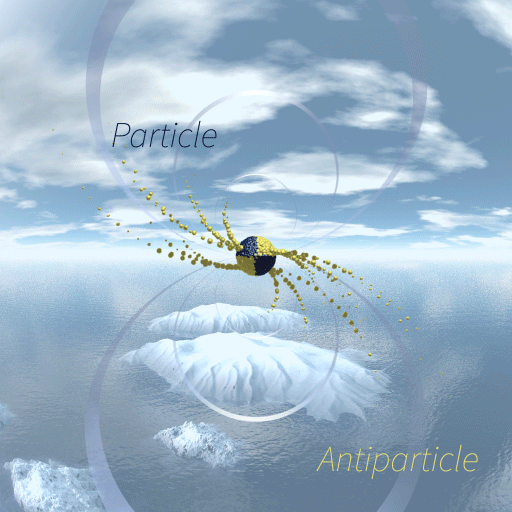Having seen an ( almost finalized ) animation for Magnetism above ,
we see the reason for little Antimatter particles moving with
superluminal speed by firstly , that Antimatter gathered at the
one pole of the magnet should repel Antiparticles leaving ,
speeding those up , so that these are passing throughout
time , moving through the bended space at the ' smallest '
distance and than being attracted by the other side of the pole ,
where Matter is accumulated ...
Secondly , it should be Antimatter particles moving very fast ,
because just bending space ( like Matter and Antimatter do )
would attract common mass objects ( e . g . an apple ) also
( what is the case , but ' vanishingly small ' in comparison with
the strong electromagnetic attraction ) .
And only magnets and magnets and a magnet and ferromagnetic
elements attract each other by the strong electromagnetic force :
Matter and Antimatter might not be bound very strong within e. g.
iron or other ferromagnetic substances , what enables a magnetic force acting .
Whereas Matter and Antimatter might be constantly separated within a magnet ...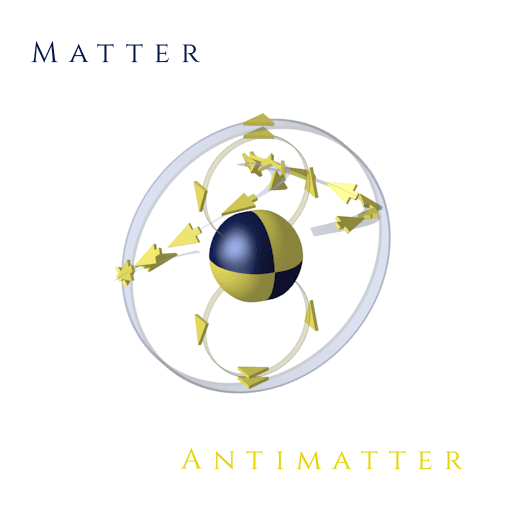At these days ( end of March of the Year 2023 ) , it seems that we have been able
to finalize our work about the ' Smallest Circular Flow of Magnetism ' ...
We might even think about an ' octopole grid ' at this times ,
instead of the current quadrupole which follows the logic of Matter being neutral
to Matter , Antimatter pushing off Antimatter . And , following from this ,
the logic of the electromagnetic attraction between Matter and Antimatter ...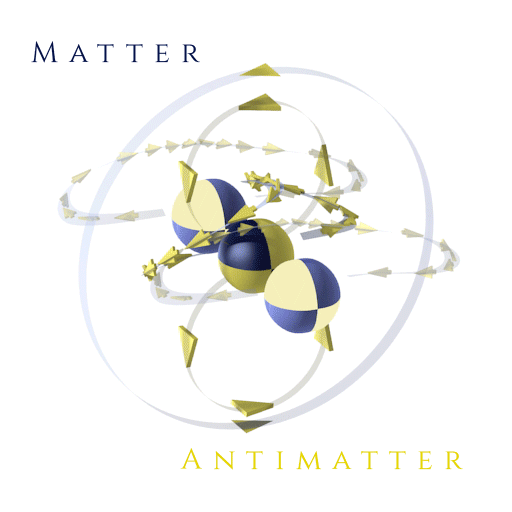We are going to see , that an octopole grid would work out fine .
While we can understand the Antimatter and Matter within this
new visualisation as single particles , because regarding atoms ,
we will see , that the characteristic of a single atom ( in case of
an element ) describes the characteristic or nature of the whole
object it is made of . Growing the mass or just size of a magnet
should increase the magnetical force , as force should add up or
even multiply ...
We will also see , that the spread and common description of the
magnetical force field up to this day might not be true . Because
The Southpole attracts the Northpole vertically and if we would rotate
one magnet of this attracting pair of magnets for 180 degrees around
this vertical axis , the result is a repelling force ...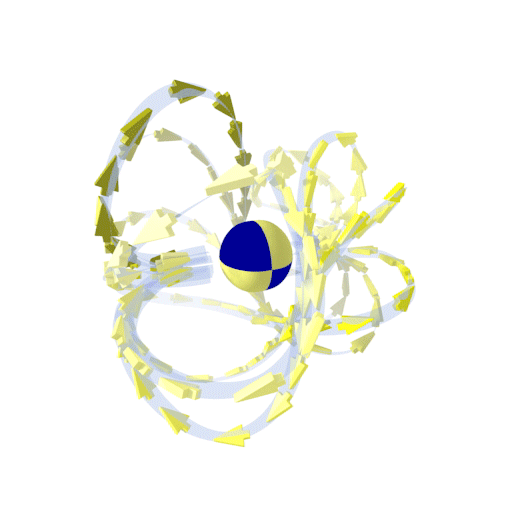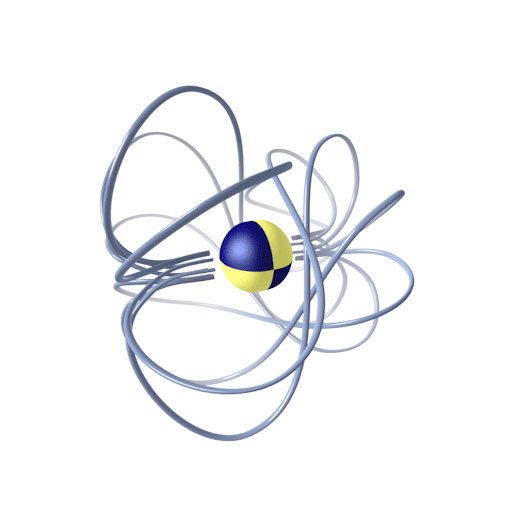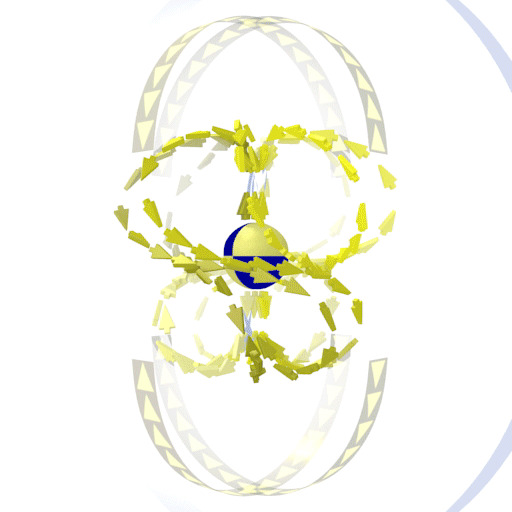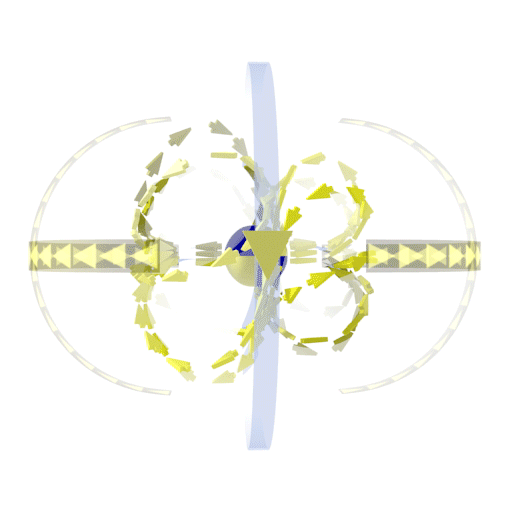Projected on a flat plane results the direction ( Northpole to Southpole )
of the ' up today common known ' magnetical force field ...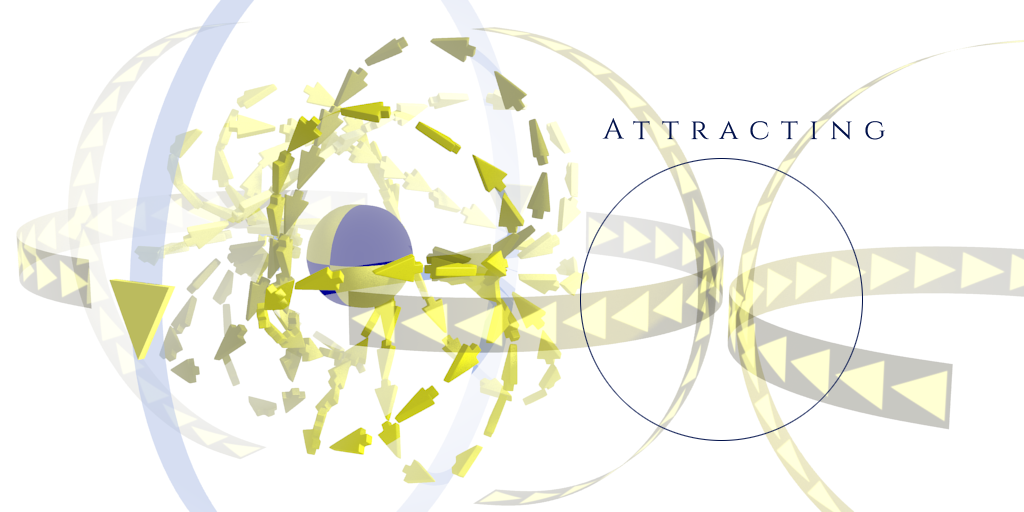Two magnetical force fields attracting each other ...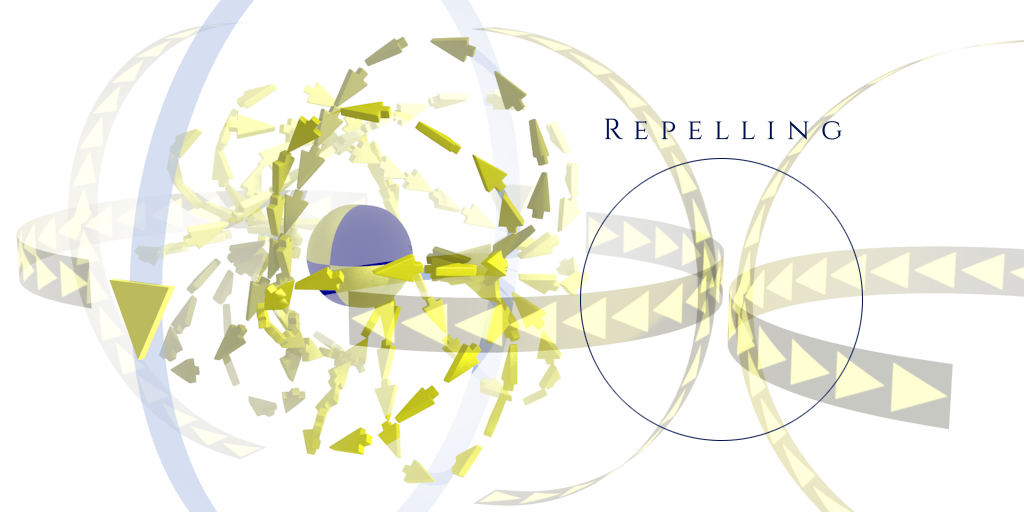Two magnetical force fields repelling each other ,
one magnet has been rotated for 180 degrees around
the axis running from ' Northpole ' to ' Southpole ' .The simplified movement from ' Northpole ' to ' Southpole ' .Matter and Antimatter distributed in harmony within common mass objects .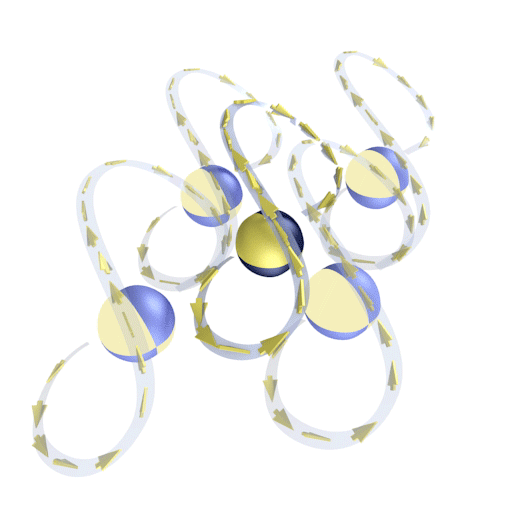The distribution of Matter and Antimatter within a magnet ( = polarized spread ) .
Electromagnetic attraction deriving from assumed superluminal
Antimatter particles might add up ...

Below we are going to see a new animation for the bending of time
by superluminal motion , while the fourth dimension , time , is reduced
to a two - dimensional area in this graphic ...
Assuming our observable Universe to be not even a dust grainlet
within the whole of our Existence ...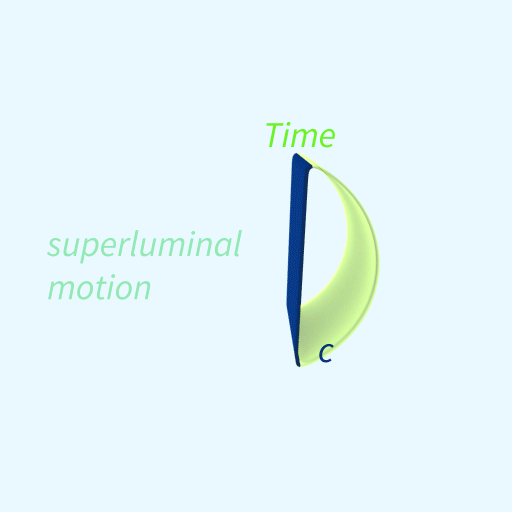The standstill of time by moving with   c   is able to show the curvature clearly ,
meanwhile the superluminal motion of antimatter might mean a travelling
throughout time and if time shrinks , the bending of space would increase .
We can just imagine a cube representing time ( reduced by one dimension )
and a ( circular ) plane within the time cube , whereas the plane represents our space
( reduced by one dimension ) . Squeezing the time cube might bend the circular plane ...
So , electromagnetic particles like photons might move circular at a large
scaling , following the natural curvature of space , meanwhile ' photon shells '
leaving a magnet move within the ' Smallest circular flow of Magnetism ' ,
as space is bended proportionally to the speed higher than   c   ...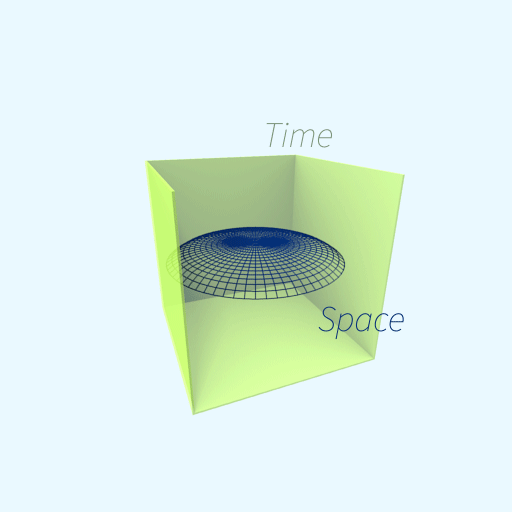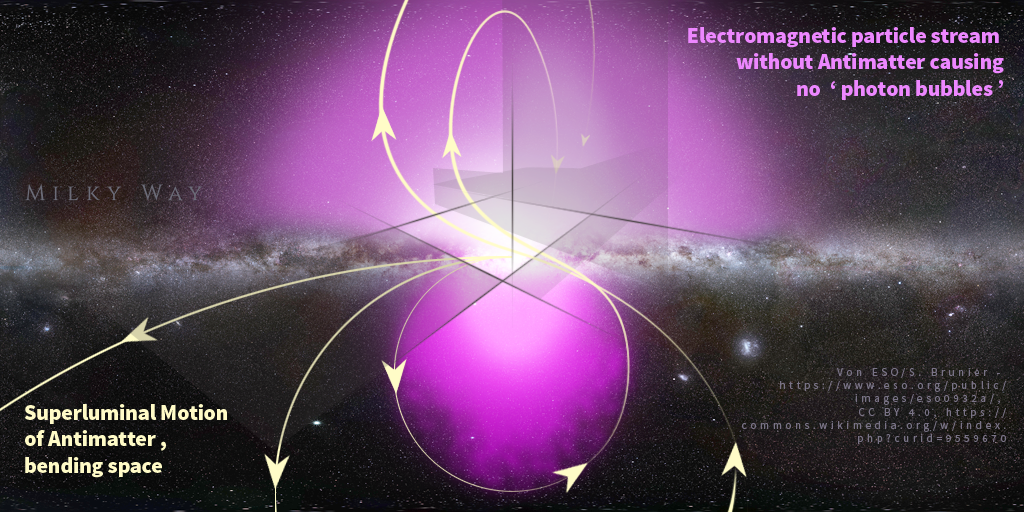After some few days to re-think all of our recent understanding ,
we might have found to the conclusion , that the high Energy in
the centers of Planets like our Earth or just Milky Way , which
should to be caused by the very large amount of gravitational
pressure causing high temperature ( = Energy ) , splits Anti -
Matter off the photon shells what is the reason for the magnetic
force field . After being just not sure , whether a magnetical field
is described by an ' 8 - like shape ' or just two circles ( both the
shapes are very similar to each other ) , we have sketched some
kind of a new understanding of the smallest circular flow of Anti -
Matter Shells of a magnetic force field ...

An issue , which has not been solved until this day , is that the
magnetic field of our Earth is like a ' laid eight ( oo ) ' compared
to its rotation axis beside the magnetic field of our Galaxy , Milky
Way , which might to be rotated for ninety degrees ( like an 8 )
according to the photon particle bubbles ...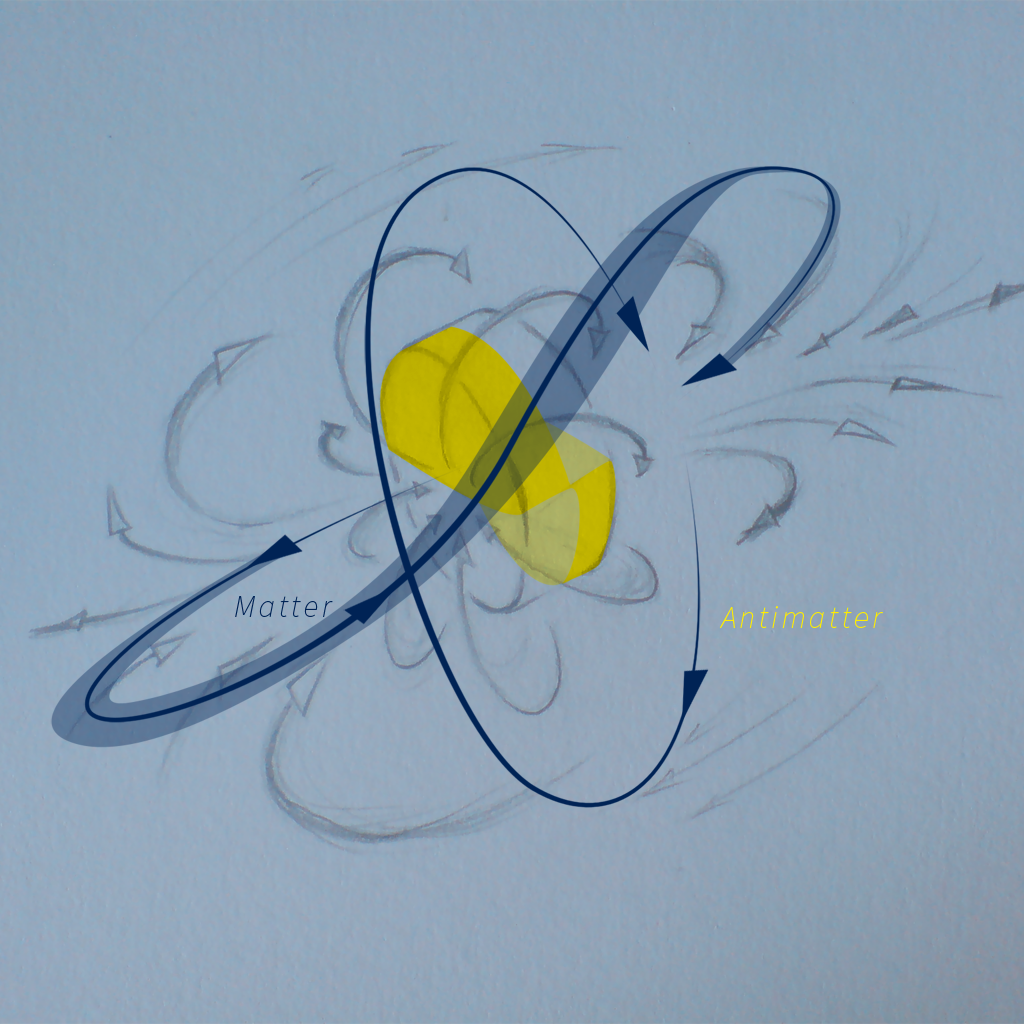Matter and Antimatter within Magnetism :
The animation above illustrates the principle of Magnetism ...
Another proof for Matter to be neutral to Matter might to be :
Within Magnetism we have two states , repelling and attracting ,
but three possibilities of combinations of the components ,
matter to matter , antimatter to matter and antimatter to antimatter ,
so one of these combinations must act as a neutral force to keep
the repelling and attracting forces equal to each other ...
This Matter - Antimatter Modell might also be a prove for the Force
of Gravitation to act only attracting ( and not repelling ) ...

After yesterday , we have again found to another , new conclusion
for the directions of a magnetic force field . As we already know ,
two magnets attract each other vertically and rotating one of the
magnets around this vertical axis results a repelling force .
It is still an open questions why the magnetic force field of our Earth
is rotated for ninety degrees compared to our idea about the superluminal
moving Antimatter split off from the center of Milky Way ...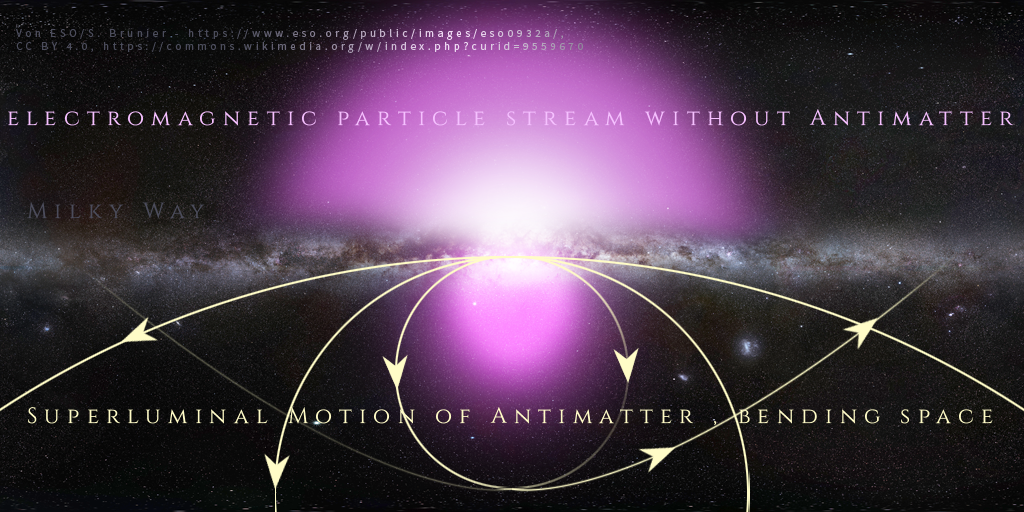We might suggest the following recognition .
Superluminal Antimatter deriving from the
high energy in the center ( photon shells
are split off their cores ) might shape both
the gamma particle bubbles and a flat disc
of our Galaxy Milky Way by passing through
the curved or bent space ...

This refined understanding might also be a proof
for the wrong assumption of the existence of dark
matter and that this kind of matter is not reality ...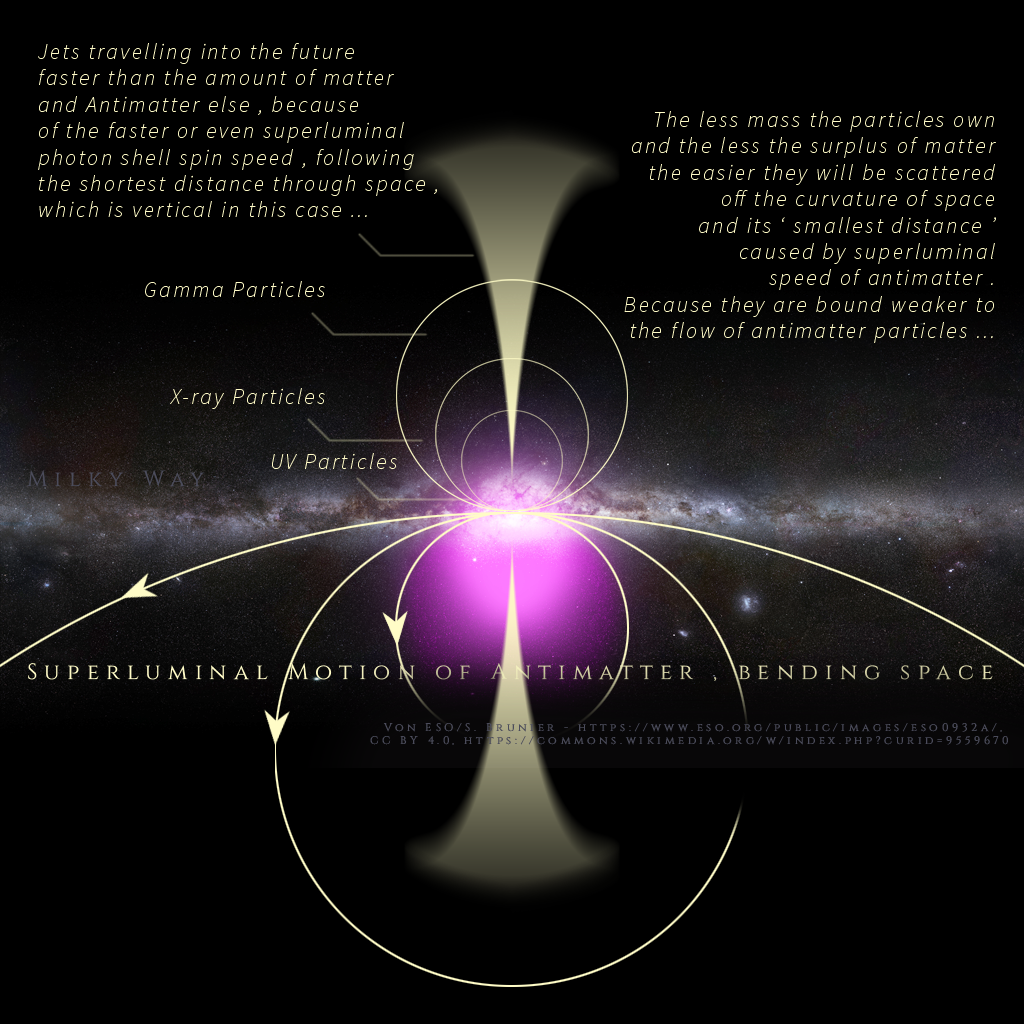During the last days we have found to the value of Energy
a photon carries , for which the Backward Causility of the
Photon Shell might to be reversed , since the photon shell
is spinning superluminal in this case and we start ahead
with this way to calculate the frequency of the photon shell
spinning with light speed c , moving right into the future :

' delta radius exponent 3 = delta frequency squared '
and because we calcuate with the value c , we can
use ' 1 / radius exponent 3 = frequency squared '

radius r = 1 / 3rd root of ( frequency f squared ) = 1 / 1,5th root of f ;
Spin Speed of the Photon shell = 2 r * pi * f = c in the case of
reverting Backward Causility ;
1 / ( 1,5 th root of f ) * f = c / 2 / pi I to the power of 1,5
f = 0,5 th root of [ ( c / 2 / pi ) to the power of 1,5 ]
f = 108.623.177.716.518.437.266.722,7158848 ;

It might be possible to measure this certain frequency and to
see the movement into the future for Antimatter in this case ...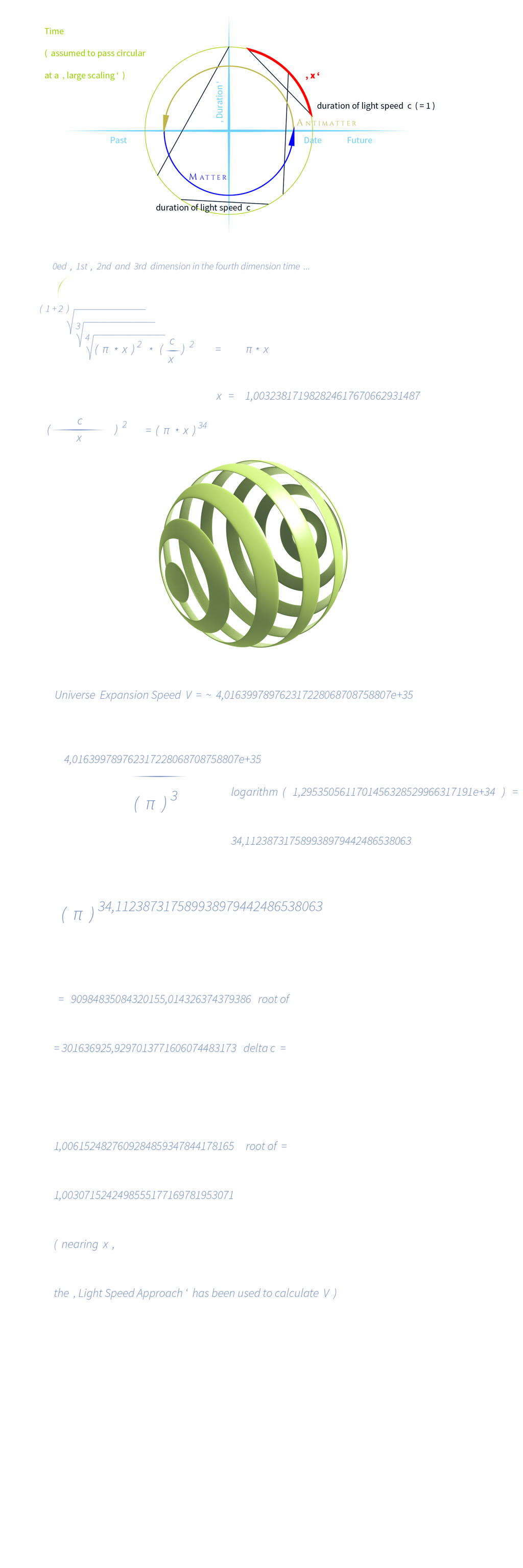We would like to finalize our work about the circular
bending of time . The factor ' x ' results speed ,
meters per second , as a deviation from one infinitely
small time unit since the time circle is projected to a
single point by extracting the 36 th root of .
In order to calculate the diameter of the circular arched
time , which is the fourth dimension , we got to expand
the calculated radius of the ' two - dimensional -
time - circle ' by its square to the power of three , since
the fourth dimension time is flattened to a two - dimensional
area and what should up in the units
meters exponent 4
per
1 second exponent 4
,
what we might understand as time and that could be the
way of converting speed to time , since a meter exponent
4 might represent time ( and a time unit exponent 4
should stay one time unit , because 1 to the power of 4
results 1 ) , but we have to know speed ratio by having
speed just to the power of four ...
The circular arched time might represent the Big Bang , our
Universe expanding and massing , pulling together again ...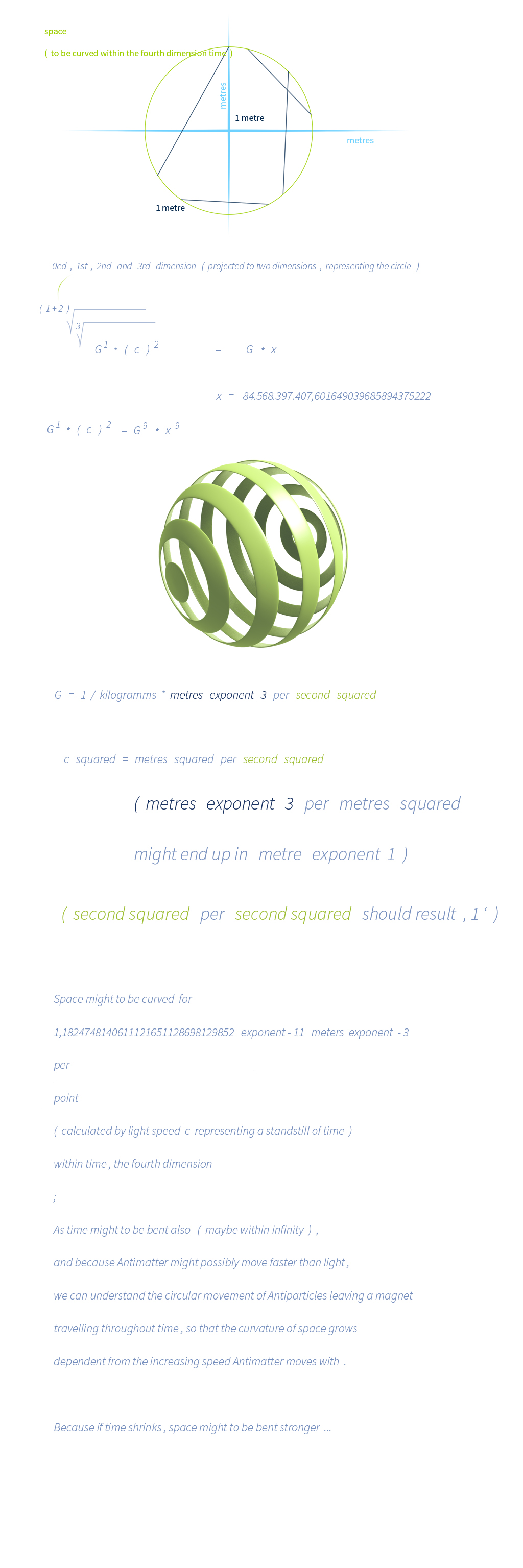Because we calculate the deviation per point by extracting the 9th root ,
we have to expand our result of meters exponent minus 3 per point
for the variable ' x ' to the power of four , resulting meter per meters
for the natural bending of space arched by the ' circular bent ' time ...

And the reason why we are going to extract the ninth root of our three
dimensions of space is , that we imagine an infinitely small cube to be
the point , and extracting the third root of this three dimensional point
results a tiny line as close to the point as possible , the difference might
to be vanishing less , so that we can set this tiny line equal to the point .

Expanding a point to a tiniest , three dimensional cube might result a cube
to exist with nine dimensions [ ( 1 exponent 3 ) exponent 3 ) ] .

We would like to add a tiny detail to our latest calculations .
Since we use a similar approach for calculating the circular
motion of time , we see the ratio of c / 1,00323817 ... ,
and that c is equal to zero time within this way to calculate ,
so we have to add the value ' 1 ' to 0 in order to receive
a ratio . Using G instead c , we might add 1 to the ratio
1 / 84.568.397.407,601649039685894375222 , so we can
per
( 1 + 1,18247 ... * 10 e -11 meters exponent - 3 )
per point

During this weekend we have found to some reasonable
explanations for calculating the natural space curvature
by using the value of the Gravitational Constant ' G ' :
' G ' is the constant of proportionality used to determine
the force two mass objects attract each other . Attracting
means bending space ( and time ) .
Since time should to be curved ( circular ) , within may
it to be infinity as the fifth dimension , space has a natural
curvature , too . And the maximum of speed for matter ,
c , might be able to show this bending exactly because
speeds faster than c would curve space , like magnetism .

We ' ll see that extracting the 9th root of metre exponent 3
results a value as close to a point as possible . A point is always ' 1 ' .

9th root of
[
G * 1 kilogramm * 1 kilogramm * c * c
/
1 metre squared ( the radius )
]
is equal to 1 ;
The point = 1 ;
1 = 1 ;
G * x ( x = the bending factor ) = 1 ;
Because G = metres exponent 3
per
kilogramm times second squared ,
and because we divide per the radius
and multiply with c squared
we are going to see that this value results
the 9th root of metres exponent 3
what is the point .
And to achieve the value for this point by
' G * x '
we have to extract the 3rd root of a meter
( or this infinitely small , three dimensional point ) for ' x ' ,
what is similar to the ' reciprocal value '
of 1 meter or just the
3rd root of this meter
or this minimal three dimensional point ,
like an ' inverted ' dimension , space or point
for the ' minimal bending ' ;
The balancing factor for G should to be x .
' G * x ' :
We extract the 9th root of metres exponent 3
what should result a value as close to a point
as possible for
( G * c squared per radius squared )
and for x
we are going to see the ' reciprocal value '
of a meter , because we extract the 3rd root
of this ' three dimensional point ' .
Possible might to be also :
Metres exponent 3 ( for G )
times
metres exponent minus 3 ( for x )
is equal to
1 ;

And multiplying with c squared should be due to the minimum
of each of the kilogramms squared have to be expanded to its
maximum force by moving with light speed c .

Calculating the circular bending of time might work out the same way ,
we are going to see the circle reduced to a point . We are using light
speed c squared due to the fourth dimension time to be flattened to
a circular plane , meanwhile c represents the diameter of this plane
in ( almost ) zero dimension of size ...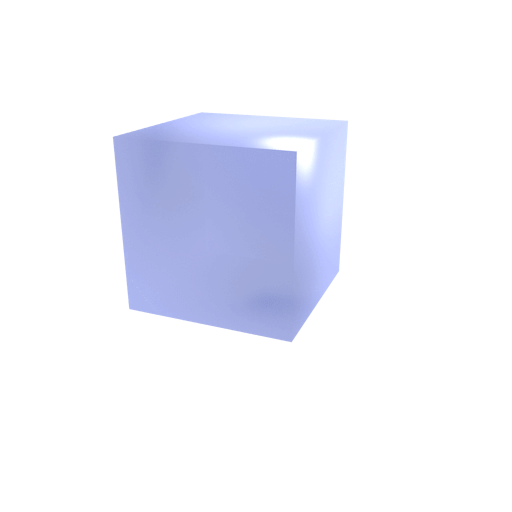The ( circular ) curvature of space can be explained by the following :
We imagine a cube as space and the movement from one point to
another one as a line within this cube representing space .
A line is always one - dimensional ...
For now , we are going to flatten the cube into a two - dimensional
plane and we think about that it is possible to wrap this plane around
a sphere , representing the bending of space .
This would make clear , that starting to travel from any point within
the spherical wrapped area we are going to reach exactly the same point
by travelling a distance which is long enough –
what is described by and in harmony with the spherical shape ...
The line ( the distance passed by ) would end up in a circle with a ' large '
diameter , whereas start - and end point should to be the same ...

It has been scientifically proven , that mass distorts time and
for now we think that this distorsion is very less because of
mass consisting of Matter and Antimatter both with a very tiny
surplus of Matter .
Matter should increase the amount of time passing what can
be seen by large celestial objects like black holes with a high
density whereas
Antimatter should invert the scale time is passing , and
we have seen that Antimatter might to be able to move faster
than Light Speed c what might to be a reasonable explanation
for the amount time is moving slower because the superluminal speed
Antimatter is able to move with might enable a flexible passing
throughout time , the fourth dimension ...

To build upon the proof of the distorsion of time by mass :
If we flatten space to two dimensions and use time as the fourth
dimension to be the third dimension , we can imagine a circle
which is passed by a line , time , as the third dimension , and
this line would pass the circle without being obstructed from ,
but with a tiny difference of proportions of 3rd dimension per
2nd dimension : Let us stay with the ' empty ' space of the
second dimension to own a mass of 1 and the circle , may it to
be our Earth , has a Mass of 4 , we are going to see a time per
mass ratio of either 1 time unit per mass or 4 time units per mass
and 4 per 1 is more , so we are right here , in the future .

The higher the energy an average electromagnetic particle holds ,
the higher the ratio - value of Matter per Antimatter what might to be
proven by the work about photons as average electromagnetic particles .
In black holes , we have a very high amount of energy because of the
large gravitational force due to lots of mass compressed to high density .
That means that matter is of more surplus than anywhere else , hence the
more we get to the center of a black hole , the more we are in the future .
The proportion of Matter and Antimatter within the core of a black hole should
not be an issue of distance between Matter and Antimatter like it is
for electromagnetic particles in free movement through a ( not perfect ) vacuum ...

There is a like to suggest a possible way to determine a ' Hubble Constant '
referring to a photon at one spin per second , while the photon shell attracted
by the core might to be compared with just a galaxy attracting another one ,
because Matter attracts Antimatter and vice versa ...

The differing measured values for the Hubble Parameter
might depend on the differing masses of e . g . galaxies ,
attracting each other by varied forces – what we are going
to see by the calculation of speed of our Moon from Earth ,
which we are going to see below ...

We are going to assume the expansion speed of space and hence force
to be almost in balance with the force of electromagnetic attraction
within single electromagnetic particles , what should be mentioned firstly .
Because if the expansion speed of space would be less ,
an electromagnetic particle would stuck together ( ' relatively fast ' ) .
If the expansion speed would be higher and let float the photon shell
off the core , we might measure red light also –
for blue light passing very high distances in space and our Universe ...
An electromagnetic shell spinning with a higher radius at same
spin speed results a lower frequency , because the shell has to pass
a higher distance for one spin , what is seen as ' red light ' , too .
This phenomenon might to be possible , because of the shell floating off
the core by expansion of space , if so , and we might to be able to
measure it by seeing the amplitude of the linear wave of the spins ...
We are going to assume an electromagnetic particle not to loose energy
during time by moving in a perfect vacuum , or just very less ( what we
will see by calculating red shifts ) ; with the comparison of our Moon
turning around our Earth in steady motion .

We are going to see , that there is no perfect vacuum in space ,
and each of the light particles has to pass a medium in some kind ,
what might to be responsible for the red shift over high distances .
the expansion of space in between the shell and core increases ) .
We have seen , that the amount of Matter shrinks like the amount
of Antimatter does by adding Energy to a photon , what means less
of electromagnetic attraction .
If a higher expansion of space would happen , the outer shell of a
photon might ' leave ' the core easier by adding energy , so we are
going to assume the measured expansion speed of space , ' v '
( while we should notice the difference between ' V ' , uppercase ,
the speed our Universe expands with , and ' v ' , not uppercase ,
which is the actual speed , two celestial bodies float off or near )
between the electromagnetic shell and its core to be
E ( Big Bang ) = ( 1 / 2 v ) squared =
= force of electromagnetic attraction between the shell and the core ...
Our equivalent for the calculation ( with a balance of speed of expansion
and strength of electromagnetic attraction ) is going to be :

1
/
{
1 Mpc ( megaparsec ) * [ ( 1 / 2 v ) squared * pi ( because we have one spin which is circular and a linear distance for space expansion ) ]
/
root of [ ( 1 / 2 v ) * pi ]
}
=
( 1 ) ( = radius of an electromagnetic particle with 1 spin per 1 second = 2,3943600123792969381313194102613e-30 meters )

If we isolate v , we are going to receive
v exponent 3 = 8 / { 1 Mpc squared * ( 1 ) squared * pi }
what results
77.556,828414033025100091639169999 metres / 1 second / 1 Megaparsec
for the expansion speed ' v ' of two celestial objects ( may be Galaxies , too ) ,
made of positive and negative mass both and attracting each other like the special
case of a photon at one spin per second ...

By using the new Hubble Parametre , we can calculate
the speed of our Moon leaving the orbit of our Earth to
0,03047295454400178969835560820641 meters / year .
Current measurements lead to 0,038 meters per year .
The average distance ( 384.400.000 meters ) of Moon
from our Earth is used , and we are going to calculate with
384.400.000 meters / 1 megaparsec
( 1 megaparsec = 3,08567758 exponent 22 meters ) *
* 77.556,828414033025100091639169999
meters per second ( / 1 Megaparsec ) * 3,154 *
10 exponent 7 ( seconds per year )
what should result the speed value mentioned above ...

So we will see , that the Hubble Parameter can vary ,
depending on the speed ' V ' our Universe expands with
(
calculated by the ' Antique Approach ' :
V = c exponent 4,200150305 ... =
4,0171778632092185994253809608252e+35 meters per second
)
what should to be a constant , while the mass of celestial
objects differs , and hence , force of attraction does ...

If the Hubble Parametre would change during time , electromagnetic particles
would have changed in their diameter also , if seen from the Big Bang ...

After some time to think about , we were able
to precise the delta , pi for the circular bent
time should to be interpreted correctly now ,
we might see about that below :

Yesterday , we have been able to calculate the
circular motion of time by our this year ' s work
to
57,388471329696442516605460751096
for the radius of the circle reduced to the point .

We have to expand this radius by its square
and then to the power of 3 because we want to
see the fourth dimension which was flattened to
a two - dimensional area what results
35.723.001.265,983871708607141433766
with a diameter of
71.446.002.531,967743417214282867532

Now , we are going to see a new , interesting part
of this work :
We assume the circular bending of space to be
stronger , if time has not passed ' half ' of
its circular motion ...
We have calculated the arch of space with
G =
{
root of ( Ge by the Planck Approach * 1,9877 ... ) =
6,7071967560044286090261498912392e-11
}
to
84.491.410.297,450656892817821774003
When we have this value to the power of five
( because we have one dimension reduced
to a point and we would like to see all of the
three dimensions )
We will recognize a natural curvature of
4,3058886640772015463419750197868e+54 meters
for a complete circular movement within space ,
when time has passed ' one spin ' and
we are going to achieve a similar value by time :
71.446.002.531,967743417214282867533
( the complete time diameter , by years )
times
( 3600 * 24 * 365,24 )
results
2.254.602.640.156.637.639,5327689798801
( seconds )
times the speed our Univere expands with
(
calculated by the Antique Approach :
c exponent 4,200150305 ... =
4,0171778632092185994253809608252e+35
)
is equal to
9,0571398163703043846779511139099e+53
and we are going to recognize a slight delta of
4,7541373450971067088547524271844
in comparison to the space curvature of
4,3058886640772015463419750197868e+54
...
This delta should to be calculated by
pi times 3rd root of pi times 36th root of pi
because we calculate with the 4th dimension
projected to two dimensions ...
( = 4,7498097606287900355550915260048 )
( With a delta delta of
1,0009111069045729061697704739499 )

Today , 4th of November of the Year 2022 ,
we have found to the following , new
possibility in order to define the ' delta delta ' ,
what we might see below :

4 - 0,9111069045729061697704739499 =
3,0888930954270938302295260501 ;

10 exponent 3,0888930954270938302295260501 =
1.227,1371257360399247147664040529 ;

logarithm ( 2,99792458 square ) *
logarithm ( 6,7071967560044286090261498912392 exponent 8 ) =
59,428643684275356314722237699005 ;
( while 6,7071967560044286090261498912392 ... is derived from G )

pi * 3rd root of pi * 36th root of pi =
4,7498097606287900355550915260048 ;

1.227,1371257360399247147664040529
/
59,428643684275356314722237699005
/
4,7498097606287900355550915260048
=

4,3473145145627768215366686090761 ;

And this value can be approached by
10 - logarithm ( 6,7071967560044286090261498912392 ... exponent 8 )
( = 10 - 6,612328372649949222357912955204 ... )
+
0,953641405855855108784532416452
(
while
logarithm of c squared =
16,953641405855855108784532416452   |
minus 16 =
0,953641405855855108784532416452
)
= 4,341313033205905886426619461248 ;

However , a division delta of
1,0013824115678751351701386066129
remains , and we see a possible reason for this
by cut off numeric values beside we are not one
hundred per cent sure about our calculations to
return true values ...

If you like to , you might see the entire work by
reading through the following lines :

We would like to show an intermediate stage
of our work for calculating the ' delta delta of
1,0009111069045729061697704739499 ' :

G = 6,7071967560044286090261498912392   |   to the power of 8
4.095.702,2117347464387129572540481   |   logarithm
6,612328372649949222357912955204 ;
c squared = 89.875.517.873.681.764   |   logarithm
16,953641405855855108784532416452 ;
pi * 3rd root of pi * 36th root of pi =
4,7498097606287900355550915260048 ;

6,612328372649949222357912955204 *
16,953641405855855108784532416452 *
4,7498097606287900355550915260048
=
532,46813300383184574227804473114 ,
which are 3 digits ,
so we have
136 + 3 = 139 ;
139 per 9 per 5 =
3,0888888888888888888888888888889 ;
3,0888888888888888888888888888889
plus
0,9111069045729061697704739499
=
3,9999957934617950586593628387889
which is very close to 4 digits of accuracy ...

But what we target are not just 3 digits by
'
532,46813300383184574227804473114
'
but
3,0001892942192223603286722545
digits
which are calculated by
'
4 - 0,9111069045729061697704739499
=
3,0888930954270938302295260501   |   * 5 * 9
139,0001892942192223603286722545 ;
139,0001892942192223603286722545 - 136 =
3,0001892942192223603286722545 ;
10 exponent 3,0001892942192223603286722545 =
1.000,4359610507793980082026168438
'

Below we are going to see the basic work :

We would like to give a reasonable answer
for the
' delta delta of
1,0009111069045729061697704739499 '
what is written below in this message :

Because we calculate with
32 digits after comma zero
within our working environment ,
light speed c squared ,
which consists of 16 digits
and G to the power of eight which
should to be 88 digits
( 10 exponent -11 to the power of 8 ) ,
we might come along
with the following calculation :

88 + 16 + 32 = 136 I per 9
( because we have x exponent 9 )

15,111111111111111111111111111111   |   per 5
( because we have
84.491.410.297,450656892817821774003
to the power of five )

3,0222222222222222222222222222222
so that the delta delta of
1,0009111069045729061697704739499
should to be directly proportional to
3,02222 ... digits of accuracy .
I am not a hundred per cent sure
but at times , it seems to work out
nicely and if we are able to precise
this way , we are going to let us know !

...

Beside our spooky actions to Halloween , we
the natural curvatures of space and time :
Firstly , it might to be possible , that after one
second of the Big Bang , time was filled with a
million of years approximately or just millions of
years had been passed by , measured by kind
of an ' external ' clock . That is the only logical
explanation for the circular motion of time and the
large distance of the Super Massive Center from
our Earth ...
And secondly we can recognize , if time has not
expanded to its maximum , space must be
bent stronger , because it has somewhere to go .

What we have found , is that time expands
from the Big Bang since it was included in
our existence and hence Light Speed c ,
the Gravitation Constant G and also the
bending of space and time will change during
the process of an expanding ( and may it
to be massing again ) Universe ...
Both the calculations above result the
current curvatures of space and time .

The changes of c and G during our
expanding Universe should to be very ,
very ' tiny ' what we have found out
by calculating with the following way :

Currently , we have a space curvature
of
1,1824748140611121651128698129852e-11
meters per meter
and our Universe expands with
4,0171778632092185994253809608252e+35
meters per second ;
4,0171778632092185994253809608252e+35
times
1,1824748140611121651128698129852e-11
is equal to
4.750.211.646.848.736.643.305.075,1692488
of the cuvature increasing per second
' approximately ' ,
times 10.000.000.000 years =
1,4990527906659369149476424016109e+42 ,
and we can add this value to the
complete current ' Space Circle ' ,
4,3058886640772015463419750197868e+54
plus
1,4990527906659369149476424016109e+42
what results
4,3058886640787005991326409567017e+54 ;
So we will see that G and c will not change
to a very high amount and our Universe should
to be older than expected , so this would to be
in harmony with the distance of our Earth to the
Super Massive Center of our Universe ...

2021 - Autumn :
Based upon the size of a single electromagnetic particle ,
a hubble parameter referring to the characteristics
of a ' photon ' at one spin per second has been determined .
We are happy to announce that we have found out
the expansion speed of our Universe at these days !
Just get in touch with me for seeing the two ways to calculate it ...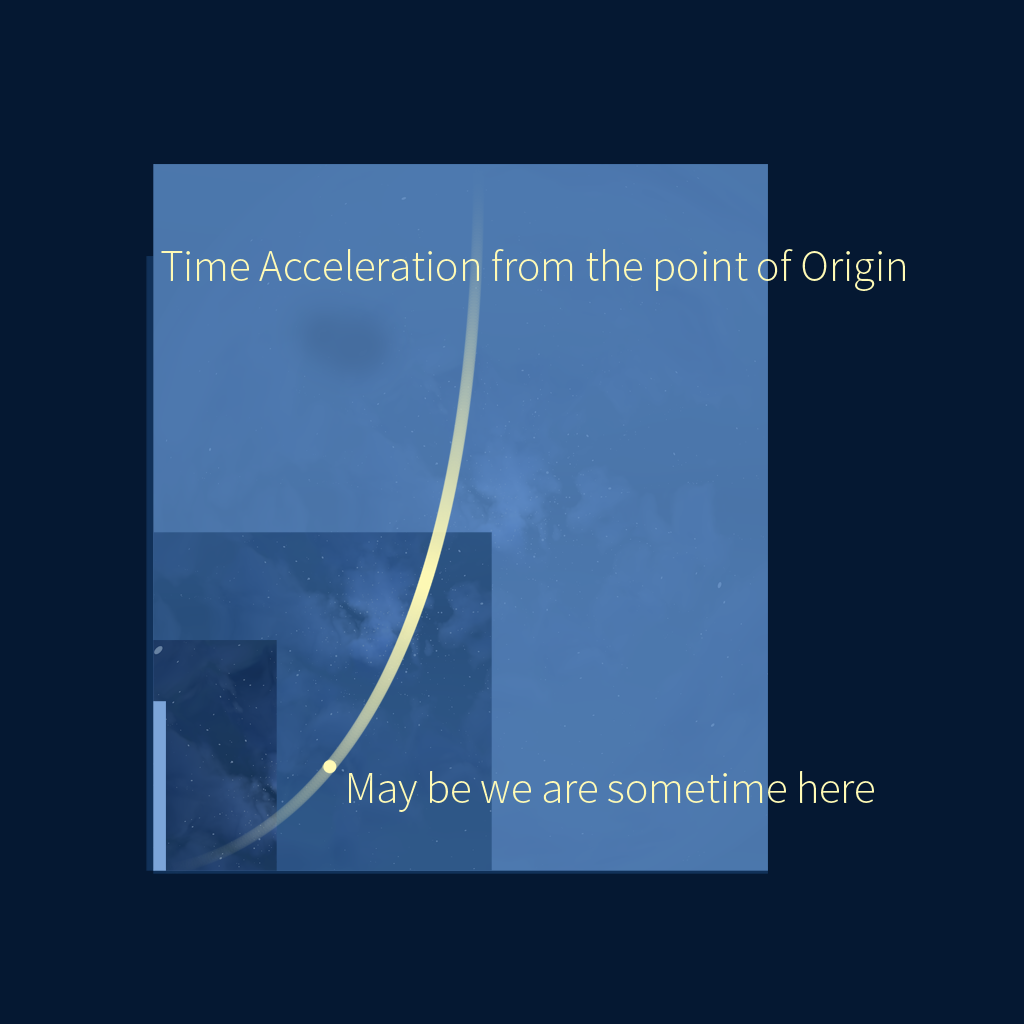Time might not pass linear – but not noticeable to us
due to an acceleration spread over a megalomaniac scale...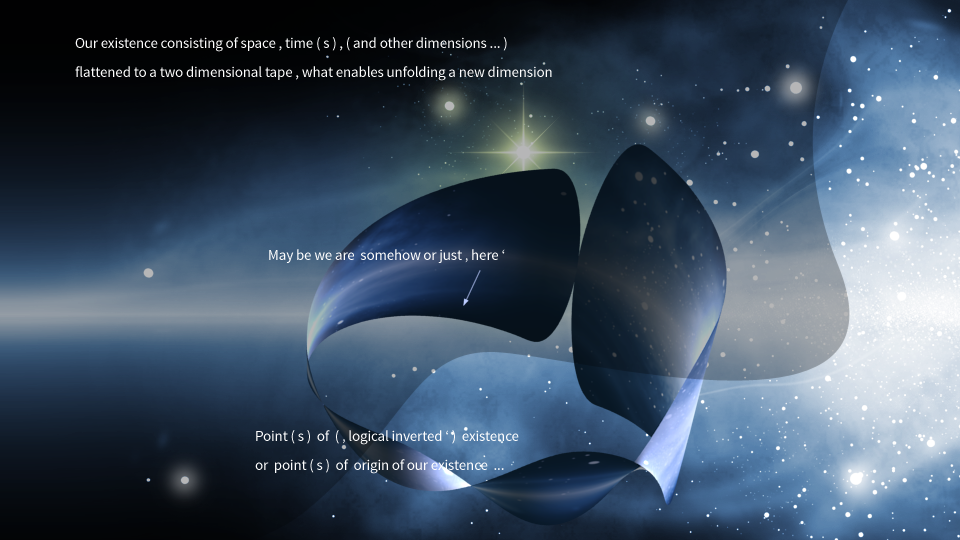Cosmos Loop Theorie ...Cosmic Infinity Time Birds , freely passing throughout time ...

' Dimension Travelling ' , understood by the three dimensions
lenght, height, depth and time as the fourth dimension
to be flattened to a two dimensional image or area –
what enables unfolding a ' new ' dimension – and assuming
some beings to own the ability to move freely within this ' new ' space ...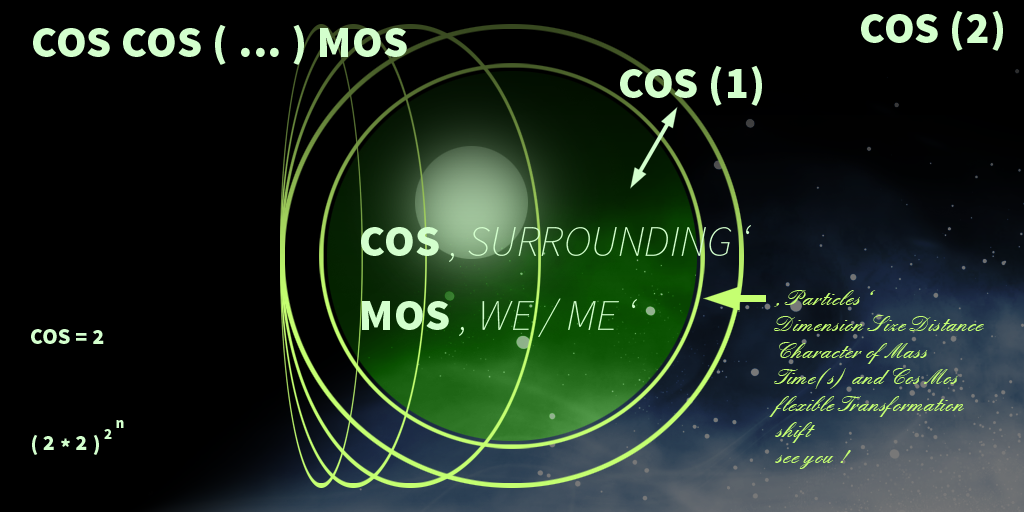Cos Cos ( ... ) Mos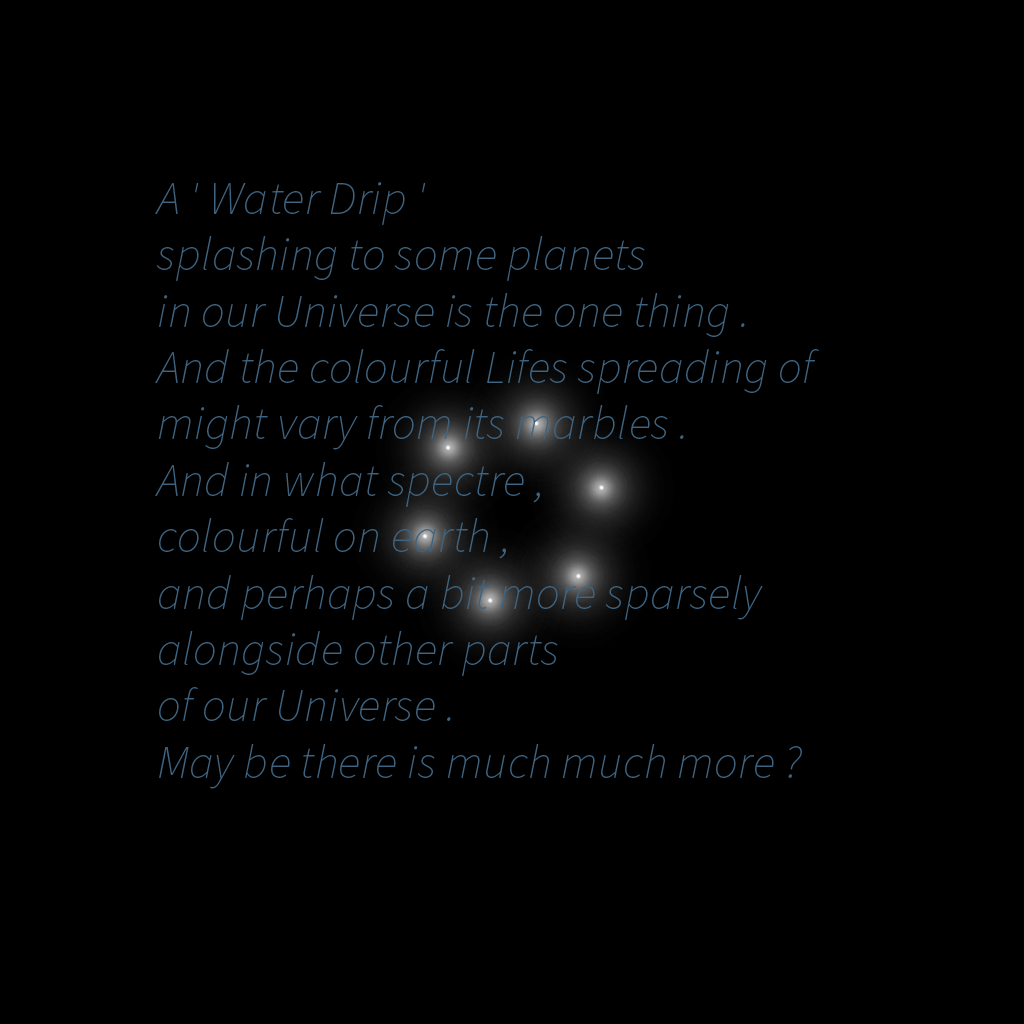Marble Water Drips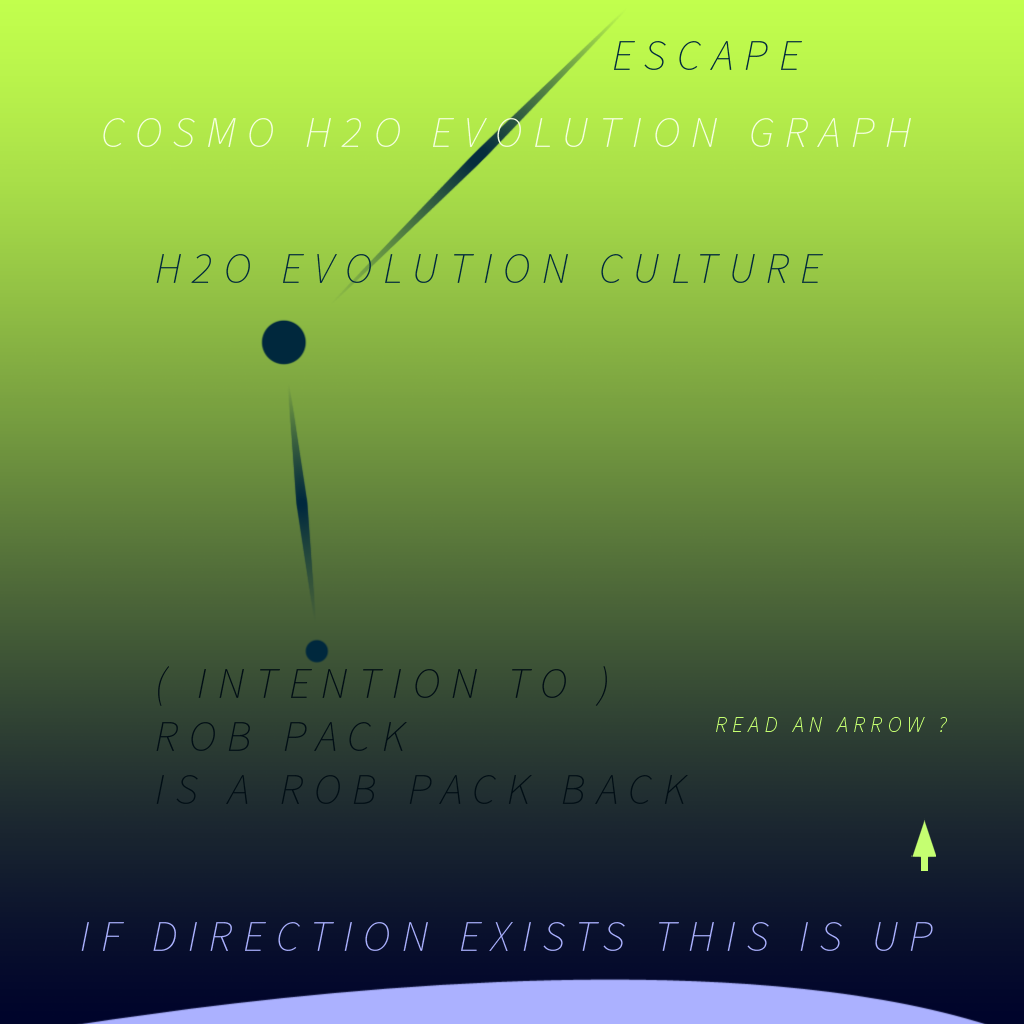Water Cosmos Evolution Graph the one Way ;)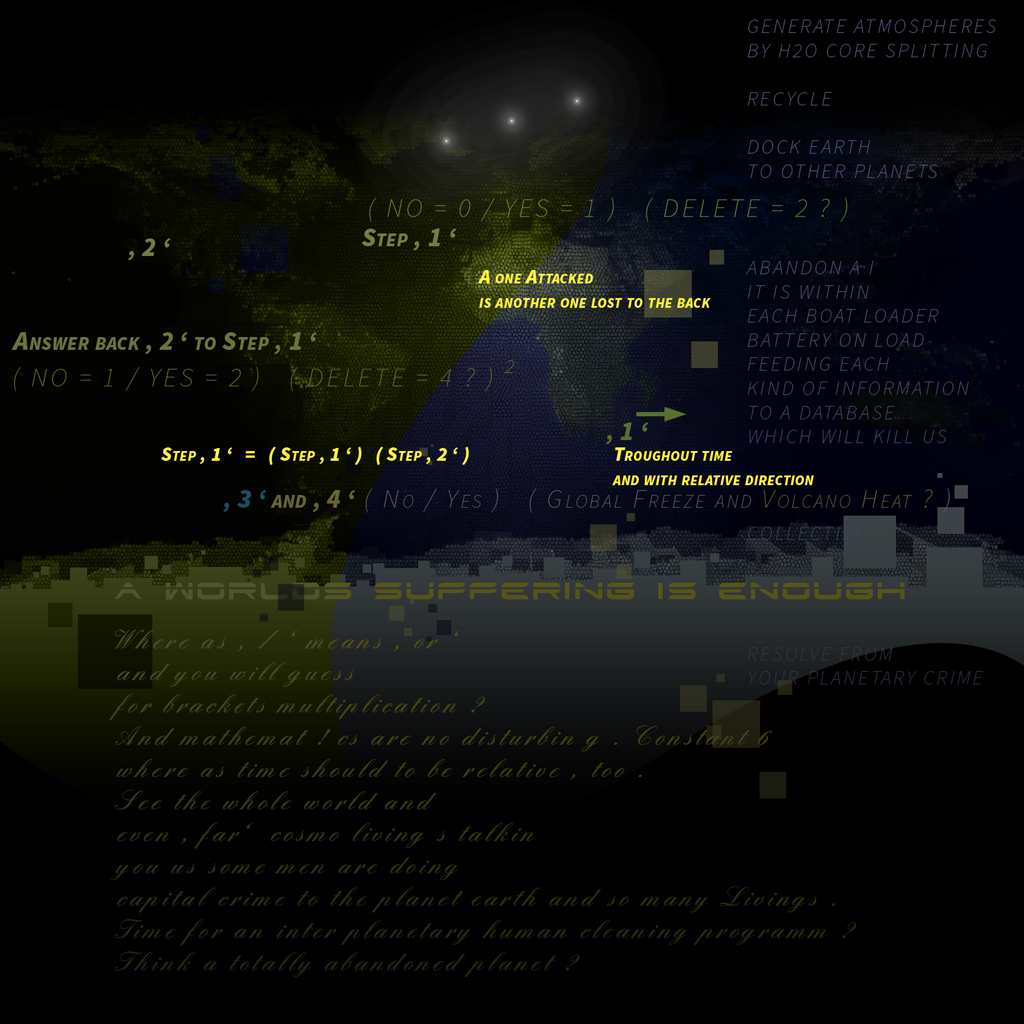A Planet abandoned ?

Cosmo Spring .

Cosmo Spring is a thought model . Named assuming an early phase of our universe .

We would like to introduce one another contribution to the world within a large sized universe
with a piece of theoretic work based on parts of existing research .
It is about to rethink the expression and complexion ' time ' and to reduce the ' existence ' ,
how we can experience it , to the most basic or simple and elementary physical,   and
mathematical sizes mass or space ( including dimension, size or distance ) , time and infinity .
Developed over a period of about eleven years , by thinking some days more and sometimes less about it ,
the video below explains the theory shortly and hopefully generelly understoodable with some additional literature .

For a better common understanding it should be mentioned that ' black holes ' actually are not
' holes ' but mass at a large concentration – it is scientifically proven , that these warp time –
and what we look at might to be liquid light around the cores. If someone would ask for ' light-particles '
we would just have asked about the gap between two things some hundred years
before , and no one would have guessed that it is filled with air – what is * something * of course.

See the video about the work here :

A ' Batch of Time ' is a visualisation here , and it should be imagined as a bunch of time ,
a little ' fluffy ' or ' unsharp ' with ' time-dust ' at its boundaries to infinity and may be
even pulsing instead of a linear or exponential expansion .

A ' ray ' actually should not exist since described as a moving point throughout time ,
which would prove that e.g. ' light-rays ' or ' radioactive radiation ' would be the wrong expression .

Time is said to move. Moving like a string it might get wavy sometime or even fluffy if understood as ' not ideal ' .
' Pulsing ' at a further perspective if thought as of infinite complexion .

Dimensional thinking:
The dimension model says that mass consists within time, and mass and time both
consist within infinity , what could to be compared with the three dimensions of space :
The 1st dimension is within the second one and 1st and 2nd dimensions
are within the third one – of mass / space what so ever called .

' Mass ' as the 1st one , ' time as the 2nd one and ' infinity ' as the third one .
And a prove might be that – if so – particles are endlessly divisible to the less and
that space ( particles / mass ) is infinite, it would be ( space and ) mass as the 1st dimension
within the third dimension ' infinity '   , near ( infinite ! / ? ) time and infinitely divisible mass –
you would guess – contained within infinity .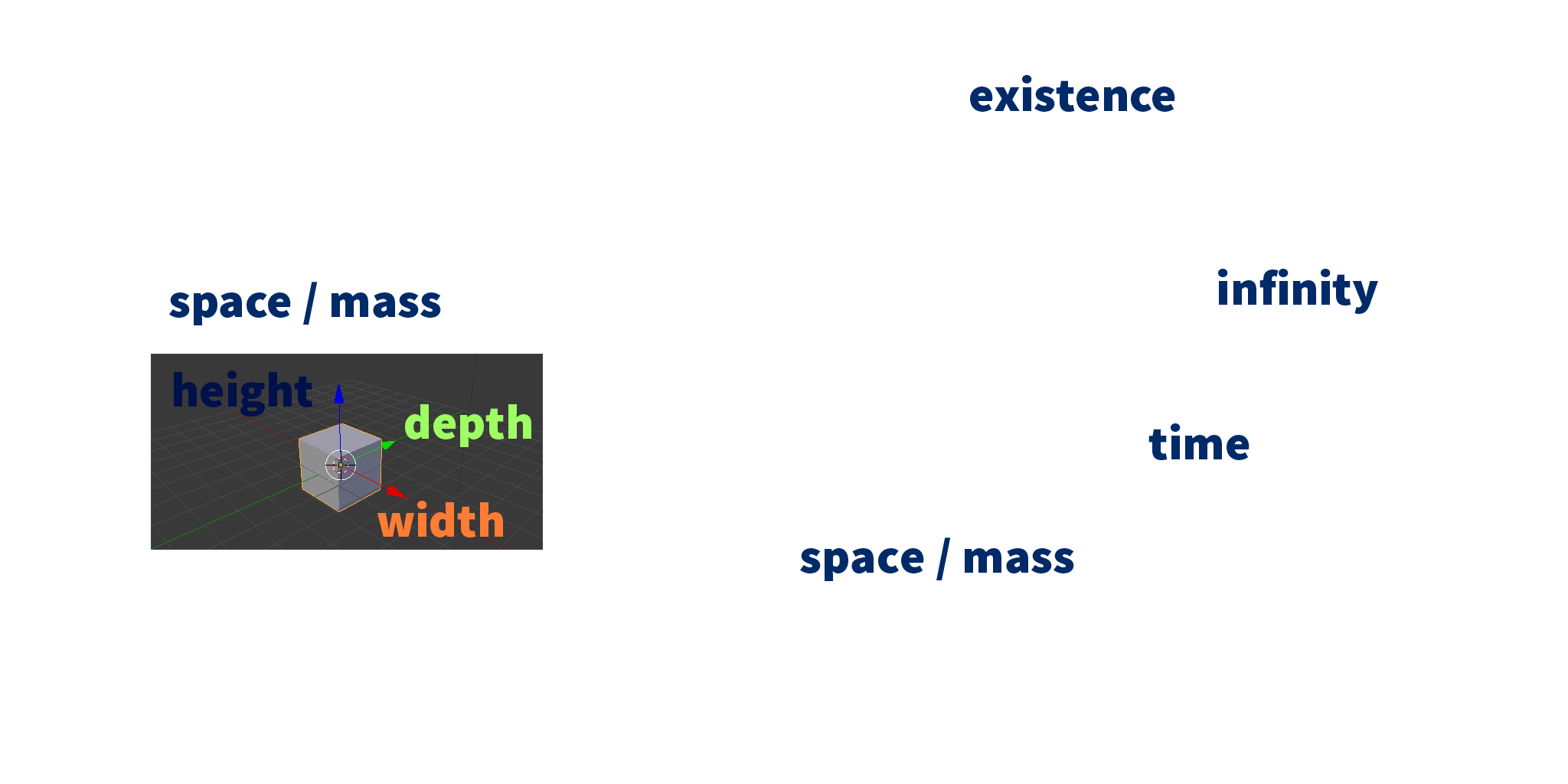With the idea to bring particle character ( reacting with each other ) to other ' existences '
which is the poetic part of the theory at the end of the video .

In some former times and only somewhere, some people believed ,
that the earth would be a plate while knowledges have been differing
by times and places .

' You can pick a thing , you might feel time , but you cannot see infinity . '

See my site for more